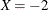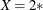### UNITS Statement

`UNITS` <independent1=list1 <independent2=list2>> </ option> ;

The UNITS statement enables you to specify units of change for the continuous explanatory variables so that customized odds ratios can be estimated. If you specify more than one UNITS statement, only the last one is used. An estimate of the corresponding odds ratio is produced for each unit of change specified for an explanatory variable. The UNITS statement is ignored for CLASS variables. Odds ratios are computed only for main effects that are not involved in interactions or nestings, unless an ODDSRATIO statement is also specified. If the CLODDS= option is specified in the MODEL statement, the corresponding confidence limits for the odds ratios are also displayed, as are odds ratios and confidence limits for any CLASS main effects that are not involved in interactions or nestings. The CLASS effects must use the GLM, reference, or effect coding.

The UNITS statement also enables you to customize the odds ratios for effects specified in ODDSRATIO statements, in which case interactions and nestings are allowed, and CLASS variables can be specified with any parameterization.

The term independent is the name of an explanatory variable and list represents a list of units of change, separated by spaces, that are of interest for that variable. Each unit of change in a list has one of the following forms:

• number

• SD or –SD

• number * SD

where number is any nonzero number, and SD is the sample standard deviation of the corresponding independent variable. For example,requests an odds ratio that represents the change in the odds when the variable X is decreased by two units.SD requests an estimate of the change in the odds when X is increased by two sample standard deviations.

You can specify the following option in the UNITS statement after a slash(/):

DEFAULT=list

gives a list of units of change for all explanatory variables that are not specified in the UNITS statement. Each unit of change can be in any of the forms described previously. If the DEFAULT= option is not specified, PROC LOGISTIC does not produce customized odds ratio estimates for any continuous explanatory variable that is not listed in the UNITS statement.